# Interest(ing) Calculator

1K+Everyone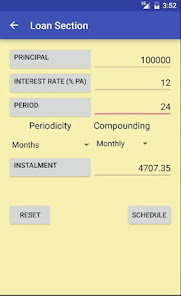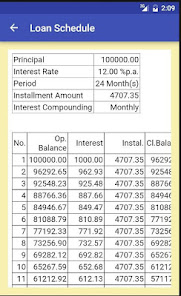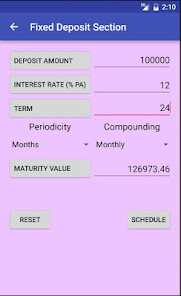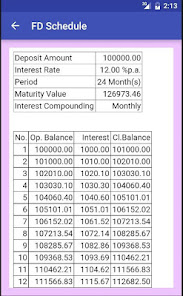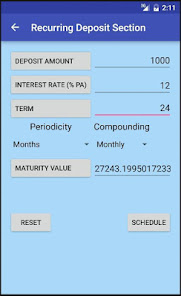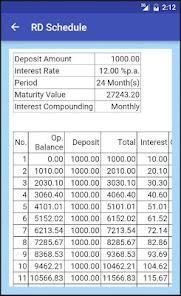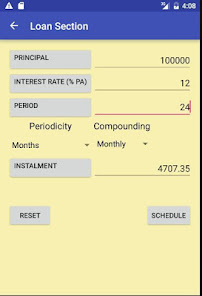Interest(ing) Calculator is a financial/interest calculator with the following features:

1. Calculate any parameter in interest calculations if you know the other three parameters. The basic parameters in financial calculations are principal, interest rate, period, installments.

2. Interest compounding period and installment periods can be selected and different. For example loans can be repaid in quarterly installments and interest can be compounded monthly.

3. Calculations for loans, fixed deposits and recurring deposits.
Updated on
Feb 15, 2016

## Data safety